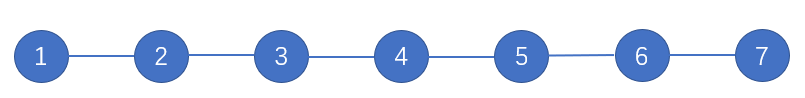【tarjan】【连通图】P3225 [HNOI2012]矿场搭建

2018-06-08 08:41:45

矿点坍塌我们可以抽象为将这个点删去。设图中原来有k个联通块，如果删掉割点，那么现在就有了k+1个联通块，如果不是割点，联通块数目不变。因此我们可以看出，割去任一个割点，割点两边的联通块都需要设一个逃生口。（关于怎么求割点）我们先来分析几个情况：

### 1.链上可以看出，图中有5个割点：2，3，4，5，6。如果不是割点的点坍塌了，例如1，那么只要在2-7中任意设置一个即可。但如果是割点坍塌了，两边的人只能往两头跑，而我们看到，在上图中，只要设置两个点：一头一尾的逃生出口，就可以满足任何坍塌情况。

### 2.环

如果在一个环上，每两个点都可以互相直达，那么我们可以设置任一个点作为逃生出口，即可满足其他所有点到达这个地方。但是要考虑逃生出口坍塌的情况，所以一个环上要设置两个逃生出口，可以使无论逃生出口是否坍塌，都有一个逃生出口可用。n个点中任选两个点，方案数是$C^2_n =\frac{n(n-1)}{2}$

### 3.一般情况

割点会分整个图为多个双连通分量，我们将每个双联通分量看作整个联通块，那么一个双连通分量中只需要设置一个点，就可以满足这个分量里的点能够跑到这个出口来。同上，我们还要考虑出口坍塌的情况。在这里，因为只会坍塌一个点，所以如果出口坍塌了，割点就不会坍塌，这个分量中的其他点可以通过割点跑到另外的双连通分量中，此时等价于同一个双连通分量。我们可以继续将这些点抽象为一个概念：叶子连通块。

### 4.叶子连通块

叶子节点是没有出度的点，入度为1，也就是只连了一条边。那么叶子连通块的概念就是：只连接了一个割点的双连通分量。  同时，对于连接两个割点的双连通分量，其中一个割点坍塌，那么另一个割点就不会坍塌，可以通过另一个割点合并到另外一个双连通分量中。而叶子连通块就不行了，如果叶子连通块的割点（叶子的父亲）被切断，那么它就是一个单独的连通块，所以这里一定要设置一个逃生出口。在这里设置一个逃生出口，有weight种选择（weight是节点数）。根据乘法原理，最小个数是叶子连通块的个数；总方案数是所有叶子连通块的weight之积。

所以我们只需要判断一个连通块dfs后是否只找到一个割点（用del数组存起来）

Code：

#include<iostream>
#include<cstring>
#include<cstdio>
using std::min;
using std::max;
using std::cin;
using std::cout;
struct node
{
int n;
node *nxt;
node(int n)
{
this->n=n;
nxt=NULL;
}
node()
{
nxt=NULL;
}
};
int dfn,low,cnt=0;//tarjan核心数组
int n,m;
bool del;
void tarjan(int x,int from)//求割点数
{
int son=0;
dfn[x]=++cnt;
low[x]=dfn[x];
while(p->nxt!=NULL)
{
p=p->nxt;
if(dfn[p->n])
low[x]=min(low[x],dfn[p->n]);
else
{
son++;
tarjan(p->n,x);
low[x]=min(low[x],low[p->n]);
if(from!=0&&low[p->n]>=dfn[x])
del[x]=true;//del=true就是割点
if(from==0&&son>1)
del[x]=true;
}
}
}
bool app;//是否出现过，判断一共有多少个点
unsigned long long sum=0;//sum<1<<64
int num=0,w=0;
bool used;
bool flag;
void dfs(int x)//判断是否为叶子连通块
{
w++;//子节点个数
while(p->nxt!=NULL)
{
p=p->nxt;
if(used[p->n])//遍历过的点或出发割点
continue;
if(del[p->n])//找到另一个非出发割点的割点，说明不是叶子连通块
{
flag=true;
continue;
}
used[p->n]=true;
dfs(p->n);
}
return;
}

int main()
{
int u,v,t=0;
scanf("%d",&m);
while(m!=0)
{
t++;
cnt=0;
n=0;
num=0;
sum=1;
memset(app,0,sizeof(app));
memset(del,0,sizeof(del));
memset(dfn,0,sizeof(dfn));
memset(used,0,sizeof(used));
for(int i=1;i<=544;i++)//最多500个点
for(int i=1;i<=m;i++)
{
scanf("%d%d",&u,&v);
app[u]=true;
app[v]=true;
if(u>n)
n=u;
if(v>n)
n=v;
tail[u]->nxt=new node(v);
tail[u]=tail[u]->nxt;
tail[v]->nxt=new node(u);
tail[v]=tail[v]->nxt;
}

for(int i=1;i<=n;i++)
if(!dfn[i])
tarjan(i,0);

for(int i=1;i<=n;i++)
if(del[i]&&app[i])
{
used[i]=true;
while(p->nxt!=NULL)
{
p=p->nxt;
if(!del[p->n]&&!used[p->n])
{
w=0;
used[p->n]=true;
flag=0;
dfs(p->n);
if(!flag)//乘法原理
{
num++;//联通块个数
sum*=w;//方案个数
}

}
}
used[i]=false;//
}
if(num==0)//如果没有割点
{
num=2;
if(n-1==m)
sum=2;
else
sum=n*(n-1)/2;//加法原理
}
printf("Case %d: %d ",t,num);
cout<<sum<<std::endl;
scanf("%d",&m);
}
return 0;
}

• star
首页